Courses

# Olympiad Test : Playing With Numbers - 1

## 10 Questions MCQ Test Maths Olympiad Class 6 | Olympiad Test : Playing With Numbers - 1

Description
This mock test of Olympiad Test : Playing With Numbers - 1 for Class 6 helps you for every Class 6 entrance exam. This contains 10 Multiple Choice Questions for Class 6 Olympiad Test : Playing With Numbers - 1 (mcq) to study with solutions a complete question bank. The solved questions answers in this Olympiad Test : Playing With Numbers - 1 quiz give you a good mix of easy questions and tough questions. Class 6 students definitely take this Olympiad Test : Playing With Numbers - 1 exercise for a better result in the exam. You can find other Olympiad Test : Playing With Numbers - 1 extra questions, long questions & short questions for Class 6 on EduRev as well by searching above.
QUESTION: 1

### The HCF of two numbers is 16 and their product is 3072. What is the LCM?

Solution:

HCF × LCM = product of numbers.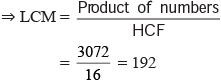QUESTION: 2

### Which of the following numbers is divisible by 3?

Solution:

2 + 4 + 3 + 5 + 7 + 8 + 0 + 6 = 35
3 + 3 + 3 + 3 + 6  + 4 + 3 + 3 = 28
3 + 5 + 7 + 6 + 9 + 8 + 1 + 2 = 41
8 + 3 + 4 + 7 + 9 + 5 + 6 + 0 = 42
Since 42 is divisible by 3 then (d) is divisible by 3.

QUESTION: 3

### Which of the following is a prime number?

Solution:

117, 171 are divisible by 3.
169 is divisible by 13.
179 is a prime number.

QUESTION: 4

Which of the following is not a twin prime?

Solution:

(23, 29) is not a twin prime.

QUESTION: 5

Find the greatest number which divides 126, 150 and 210 leaving remainder 6 in each case.

Solution: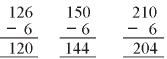HCF of 120 and 144 = 12
HCF of 120 and 204 = 12
Required number = HCF of 120, 144, 204 = 12

QUESTION: 6

Find the largest number that will divide 76, 113 and 186 leaving remainder 4, 5, 6 respectively.

Solution: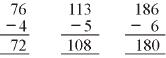HCF of 72 and 108 = 36
HCF of 108 and 180 = 36
∴ required number = 36

QUESTION: 7

Find the smallest number which when divided by 16, 36 & 40 leaves a remainder 7 in each case.

Solution:

LCM of 16, 36 and 40 is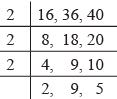∴ LCM = 2 × 2 × 2 × 2 × 9 × 5
= 16 × 45 = 720
∴ required number = (720+7) = 727

QUESTION: 8

Which greatest number of 4 digits is exactly divisible by 12, 16, 28 & 36?

Solution:

The greatest 4 digit number = 9999. Here,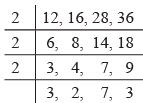LCM of 12, 16, 28 and 36
= 2 × 2 × 2 × 3 × 2 × 7 × 3
= 4 × 9 × 4 × 7
= 16 × 9 × 7
= 1008
∴ 9072 is a multiple of 1008.
∴  it is the greatest number of 4 digits which is divisible by 12, 16, 28 & 36.

QUESTION: 9

The HCF of two numbers is 23 and their LCM is 1449. If one of the numbers is 161 what is the other?

Solution:

LCM × HCF = one number × second number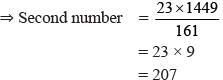QUESTION: 10

Find the smallest number which when divided by 3 is divisible by 21, 28, 36 and 45?

Solution:

LCM of 21, 28, 36 and 45 is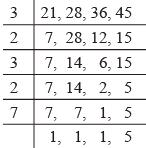∴ LCM of 21, 28, 36 and 45
= 3 × 2 × 3 × 2 × 7 × 5
= 36 × 35
= 1260
∴ Required number = 1260 + 3 = 1263